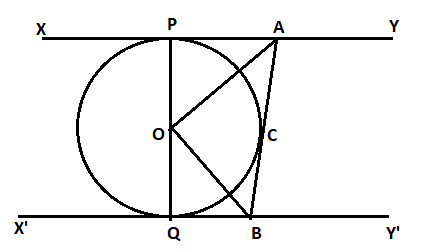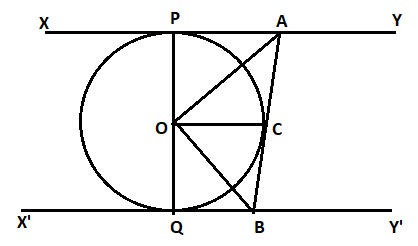QUESTION

# In the figure XY and X’Y’ are two parallel tangents to a circle with centre O and another tangent AB with point of contact C intersecting XY at A and X’Y’ at B prove that $\angle {\text{AOB = 9}}{{\text{0}}^\circ}$.Hint: Tangent at any point of a circle is perpendicular to the radius through the point of contact. We will join O to C and then use a congruence and linear pair of angles to prove that $\angle {\text{AOB = 9}}{{\text{0}}^\circ }$.

Now, we will join O to C and draw the figure.Now, from the figure, we can see that OC, OP and OQ are the radiuses of the circle. Now, as the tangent is perpendicular to radius at point of contact, so $\angle {\text{OPA = 9}}{{\text{0}}^\circ }$. Also, $\angle OQC{\text{ }} = {\text{ }}\angle OCA{\text{ }} = {\text{ }}\angle OCB{\text{ }} = {\text{ }}{90^\circ }$. Also, the lengths of tangents drawn from an external point to a circle are equal. Therefore, for an external point A, PA = PC, for point B, QB = BC.
So, in triangle OQA and triangle OCA, we have
PA = PC (lengths of tangent)
$\angle {\text{OPA = }}\angle {\text{OCA}}$ (each equal to ${90^\circ }$)
So, $\Delta {\text{OQA }} \cong {\text{ }}\Delta {\text{OCA}}$ by SAS property.
So, by c.p.c.t we get, $\angle {\text{POA = }}\angle {\text{COA}}$ …. (1)
Now, in triangle OQB and OCB, we have
BQ = BC (lengths of tangent)
$\angle {\text{OQB = }}\angle {\text{OCB}}$ (each equal to ${90^\circ }$)
So, $\Delta {\text{OQB }} \cong {\text{ }}\Delta {\text{OCB}}$ by SAS property.
So, by c.p.c.t. we get, $\angle {\text{QOB = }}\angle {\text{COB}}$ … (2)
Now, by linear pair of angles which states that the sum of all angles in a straight line is equal to ${180^\circ }$.
$\angle POA{\text{ }} + {\text{ }}\angle AOC{\text{ }} + {\text{ }}\angle QOC{\text{ }} + {\text{ }}\angle BOC{\text{ }} = {\text{ }}{180^\circ }$
$2\angle AOC{\text{ }} + {\text{ }}2\angle BOC{\text{ }} = {\text{ }}{180^\circ }$
$2\angle {\text{AOB = 18}}{{\text{0}}^\circ }$
Therefore, $\angle {\text{AOB = 9}}{{\text{0}}^\circ }$. Hence proved.